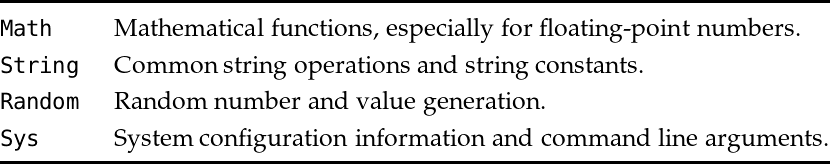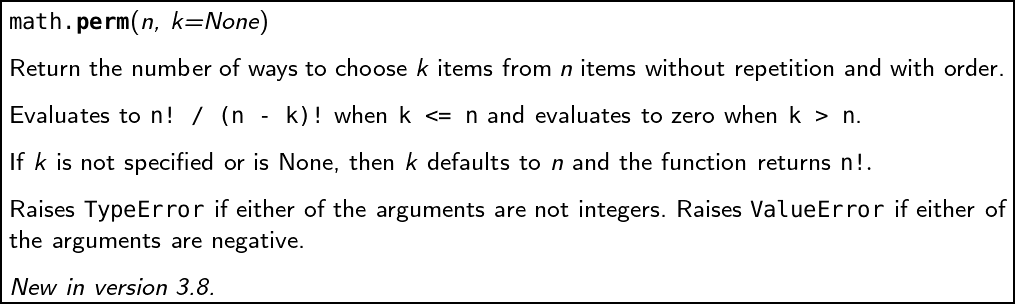Back to Contents

# 11. The Standard Library

We can divide the words and symbols we have been using to build Python programs into three kinds:

1. The language itself. For example, words like `if` and `return`. These also include operators like `+`.

2. Things which are not part of the language, but which are always available, such as `input` and `map`.

3. Things we had to ask for specifically by using `import`. These are extra modules supplied with Python, and called the Standard Library.

It is this last category which concerns us here.

### Python’s Standard Library

The Python Standard library is divided into modules, one for each area of functionality (in the next chapter, we will learn how to write our own modules). We have already seen how to use `import` statement to make available functions from a module. Here are the modules we have already used from the Standard Library:Previously, we introduced the `import` construct. Let us review it now. We can use `from` … `import` … to access definitions and functions from another module. As we know, the functions from a module can be used by putting a period (full stop) between the module name and the function. As an example, the `perm` function in the `math` module can be used like this:

``````Python
>>> import math
>>> math.perm(5, 2)
20``````

We can use `from` … `import` `*` to import all definitions from a script:

``````Python
>>> from math import *
>>> perm(5, 2)
20``````

We would not normally do this with Standard Library modules: names may clash with our own functions, leading to bugs. We can reduce this problem by importing only the functions we want:

``````Python
>>> from math import perm, factorial
>>> perm(5, 2)
20
>>> factorial(10)
3628800
>>> ceil(2.3)
Traceback (most recent call last):
File "<stdin>", line 1, in <module>
NameError: name 'ceil' is not defined``````

In any event, if we need to use a function with a long name several times, we can rename it ourselves:

``````Python
>>> import math
>>> fac = math.factorial
>>> fac(10)
3628800``````

### Example: the math module

We will take the `math` module as an example. You can find the documentation for the Python Standard Library installed with your copy of Python, or on the internet. Make sure you are looking at the documentation for Python 3, not any earlier version. Here is the Python documentation for `math.perm`:In the documentation, we are told what the function does for each argument, and what exceptions may be raised.

## Summary

We have learned how to look up functions in the documentation for Python’s Standard Library, giving us access to a huge range of modules for everything from text processing, to graphics, to internet programming. In the next chapter, we will talk more about structuring the sort of larger programs we might write using a combination of our own functions and Standard Library functions.

The questions for this chapter use functions from the Standard Library, so you will need to have a copy of the documentation to hand.

## Questions

1. Compare the `math.factorial` function supplied with Python to the one we wrote in chapter 2. How do they differ?

2. Use the `string` module to write a function which detects if a given string represents a positive integer or not.

3. The function `getpass.getpass` from the `getpass` module can be used to accept input from the user without showing it on screen, in the manner in which we might type a password. Use this function to write a version of the guessing game from chapter 3 question 7 which allows one person to set up the guessing game in front of another, choosing the number to be guessed.

4. Use the `statistics` module to calculate the median, mode, and mean of a given list of numbers.

5. Use the functions `time.time` and `time.sleep` from the `time` module to write a reaction-time testing game.